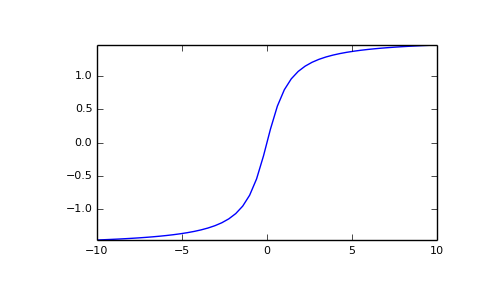# numpy.arctan¶

numpy.arctan(x[, out]) = <ufunc 'arctan'>

Trigonometric inverse tangent, element-wise.

The inverse of tan, so that if y = tan(x) then x = arctan(y).

Parameters: x : array_like Input values. arctan is applied to each element of x. out : ndarray Out has the same shape as x. Its real part is in [-pi/2, pi/2] (arctan(+/-inf) returns +/-pi/2). It is a scalar if x is a scalar.

arctan2
The “four quadrant” arctan of the angle formed by (x, y) and the positive x-axis.
angle
Argument of complex values.

Notes

arctan is a multi-valued function: for each x there are infinitely many numbers z such that tan(z) = x. The convention is to return the angle z whose real part lies in [-pi/2, pi/2].

For real-valued input data types, arctan always returns real output. For each value that cannot be expressed as a real number or infinity, it yields nan and sets the invalid floating point error flag.

For complex-valued input, arctan is a complex analytic function that has [1j, infj] and [-1j, -infj] as branch cuts, and is continuous from the left on the former and from the right on the latter.

The inverse tangent is also known as atan or tan^{-1}.

References

Abramowitz, M. and Stegun, I. A., Handbook of Mathematical Functions, 10th printing, New York: Dover, 1964, pp. 79. http://www.math.sfu.ca/~cbm/aands/

Examples

We expect the arctan of 0 to be 0, and of 1 to be pi/4:

```>>> np.arctan([0, 1])
array([ 0.        ,  0.78539816])
```
```>>> np.pi/4
0.78539816339744828
```

Plot arctan:

```>>> import matplotlib.pyplot as plt
>>> x = np.linspace(-10, 10)
>>> plt.plot(x, np.arctan(x))
>>> plt.axis('tight')
>>> plt.show()
```numpy.arccos

numpy.hypot